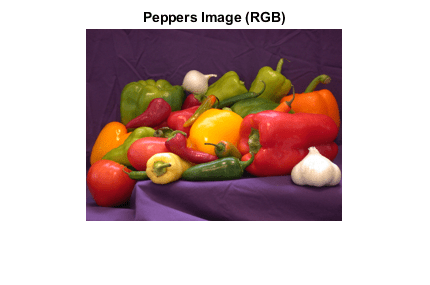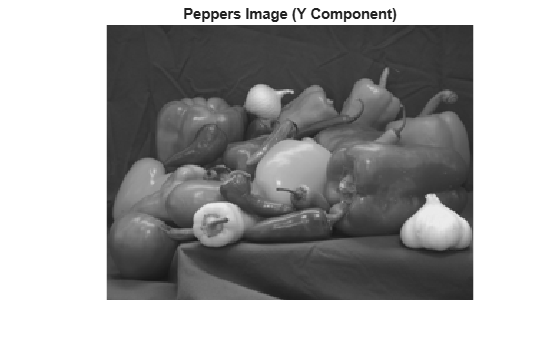# write

Write entire image

## Syntax

``write(t,imageData)``
``write(t,Y,Cb,Cr)``

## Description

example

````write(t,imageData)` writes `imageData` to TIFF file associated with the `Tiff` object `t`. The `write` method breaks the data into strips or tiles, depending on the value of the `RowsPerStrip` tag, or the `TileLength` and `TileWidth` tags.```

example

````write(t,Y,Cb,Cr)` writes the YCbCr component data to the TIFF file. Use this syntax only for images with a YCbCr photometric interpretation.```

## Examples

collapse all

Write a RGB image and multiple tag values that characterize the image to a new TIFF file.

Load the image data and display the image.

```load('peppers_RGB.mat'); imshow(RGB); title('Peppers Image (RGB)');```Create a `Tiff` object to write the image data to a TIFF file.

`t = Tiff('myfile.tif','w'); `

Use the `tagstruct` structure to define multiple tag values that characterize the image.

```tagstruct.ImageLength = size(RGB,1); tagstruct.ImageWidth = size(RGB,2); tagstruct.Photometric = Tiff.Photometric.RGB; tagstruct.BitsPerSample = 8; tagstruct.SamplesPerPixel = 3; tagstruct.PlanarConfiguration = Tiff.PlanarConfiguration.Chunky; tagstruct.Software = 'MATLAB'; ```

Set the tag values for the `Tiff` object.

`setTag(t,tagstruct)`

Write image data to the file and then close the `Tiff` object.

```write(t,RGB); close(t);```

Write a YCbCr image and multiple tag values that characterize the image to a new TIFF file.

Load and display the Y component of the image.

```load('peppers_YCbCr.mat'); imshow(Y); title('Peppers Image (Y Component)');```Create a `Tiff` object to write the image data to a TIFF file.

`t = Tiff('myfile_YCbCr.tif','w'); `

Use the `tagstruct` structure to define multiple tag values that characterize the image.

```tagstruct.ImageLength = size(Y,1); tagstruct.ImageWidth = size(Y,2); tagstruct.SampleFormat = 1; % uint tagstruct.Photometric = Tiff.Photometric.YCbCr; tagstruct.BitsPerSample = 8 ; tagstruct.SamplesPerPixel = 3; tagstruct.YCbCrSubSampling = [1,1]; tagstruct.Compression = Tiff.Compression.None; tagstruct.PlanarConfiguration = Tiff.PlanarConfiguration.Chunky; tagstruct.Software = 'MATLAB'; ```

Set the tag values for the `Tiff` object.

`setTag(t,tagstruct)`

Write image data to the file, and then close the `Tiff` object.

```write(t,Y,Cb,Cr) close(t)```

## Input Arguments

collapse all

`Tiff` object representing a TIFF file. Use the `Tiff` function to create the object.

Image data, specified as a numeric array. For example, for an RGB image `imageData` is an `M`-by-`N`-by-`3` array. Where `M` and `N` are the number of rows and columns in the image, respectively.

Data Types: `double`

Luma component of the image data, specified as a two-dimensional numeric array.

Data Types: `double`

Blue-difference chroma component of the image data, specified as a two-dimensional numeric array.

Data Types: `double`

Red-difference chroma component of the image data, specified as a two-dimensional numeric array.

Data Types: `double`

## Tips

• Writing TIFF images with certain combinations of photometric configuration and the number of samples per pixel is not recommended. The value of `SamplesPerPixel` must be equal to the sum of `Photometric` color channels and the `ExtraSamples` specified in the `Tiff` object.

## Version History

Introduced in R2009b